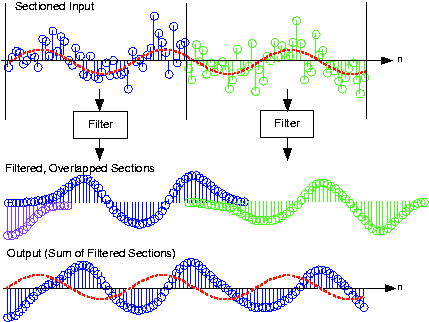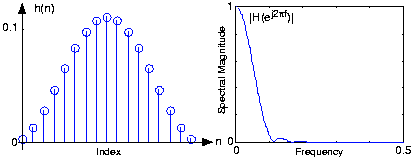# Efficiency of Frequency-Domain Filtering

To determine for what signal and filter durations a time- or frequency-domain implementation would be the most efficient, we need only count the computations required by each. For the time-domain, difference-equation approach, we need

. The frequency-domain approach requires three Fourier transforms, each requiring

computations for a length-

FFT, and the multiplication of two spectra (

computations). The output-signal-duration-determined length must be at least

. Thus, we must compare

Exact analytic evaluation of this comparison is quite difficult (we have a transcendental equation to solve). Insight into this comparison is best obtained by dividing by

.

With this manipulation, we are evaluating the number of computations per sample. For any given value of the filter's order

, the right side, the number of frequency-domain computations, will exceed the left if the signal's duration is long enough. However, for filter durations greater than about 10, as long as the input is at least 10 samples, the frequency-domain approach is faster so long as the FFT's power-of-two constraint is advantageous.

The frequency-domain approach is not yet viable; what will we do when the input signal is infinitely long? The difference equation scenario fits perfectly with the envisioned digital filtering structure, but so far we have required the input to have limited duration (so that we could calculate its Fourier transform). The solution to this problem is quite simple: Section the input into frames, filter each, and add the results together. To section a signal means expressing it as a linear combination of length-

non-overlapping "chunks." Because the filter is linear, filtering a sum of terms is equivalent to summing the results of filtering each term.

As illustrated in Figure 1, note that each filtered section has a duration longer than the input. Consequently, we must literally add the filtered sections together, not just butt them together.Figure 1. The noisy input signal is sectioned into length-48 frames, each of which is filtered using frequency-domain techniques. Each filtered section is added to other outputs that overlap to create the signal equivalent to having filtered the entire input. The sinusoidal component of the signal is shown as the red dashed line.

Computational considerations reveal a substantial advantage for a frequency-domain implementation over a time-domain one. The number of computations for a time-domain implementation essentially remains constant whether we section the input or not. Thus, the number of computations for each output is

. In the frequency-domain approach, computation counting changes because we need only compute the filter's frequency response

once, which amounts to a fixed overhead. We need only compute two DFTs and multiply them to filter a section. Letting

denote a section's length, the number of computations for a section amounts to

. In addition, we must add the filtered outputs together; the number of terms to add corresponds to the excess duration of the output compared with the input (

). The frequency-domain approach thus requires

computations per output value. For even modest filter orders, the frequency-domain approach is much faster.

### Exercise

Show that as the section length increases, the frequency domain approach becomes increasingly more efficient.

Let

denote the input's total duration. The time-domain implementation requires a total of

computations, or

computations per input value. In the frequency domain, we split the input into

sections, each of which requires

per input in the section. Because we divide again by

to find the number of computations per input value in the entire input, this quantity decreases as

increases. For the time-domain implementation, it stays constant.

Note that the choice of section duration is arbitrary. Once the filter is chosen, we should section so that the required FFT length is precisely a power of two: Choose

so that

.

Implementing the digital filter shown in the A/D block diagram with a frequency-domain implementation requires some additional signal management not required by time-domain implementations. Conceptually, a real-time, time-domain filter could accept each sample as it becomes available, calculate the difference equation, and produce the output value, all in less than the sampling interval

. Frequency-domain approaches don't operate on a sample-by-sample basis; instead, they operate on sections. They filter in real time by producing

outputs for the same number of inputs faster than

. Because they generally take longer to produce an output section than the sampling interval duration, we must filter one section while accepting into memory the next section to be filtered. In programming, the operation of building up sections while computing on previous ones is known as buffering. Buffering can also be used in time-domain filters as well but isn't required.

We want to lowpass filter a signal that contains a sinusoid and a significant amount of noise. The example shown in Figure 1 shows a portion of the noisy signal's waveform. If it weren't for the overlaid sinusoid, discerning the sine wave in the signal is virtually impossible. One of the primary applications of linear filters is noise removal: preserve the signal by matching filter's passband with the signal's spectrum and greatly reduce all other frequency components that may be present in the noisy signal.

A smart Rice engineer has selected a FIR filter having a unit-sample response corresponding a period-17 sinusoid:

,

, which makes

. Its frequency response (determined by computing the discrete Fourier transform) is shown in Figure 2. To apply, we can select the length of each section so that the frequency-domain filtering approach is maximally efficient: Choose the section length

so that

is a power of two. To use a length-64 FFT, each section must be 48 samples long. Filtering with the difference equation would require 33 computations per output while the frequency domain requires a little over 16; this frequency-domain implementation is over twice as fast! Figure 1 shows how frequency-domain filtering works.Figure 2. The figure shows the unit-sample response of a length-17 Hanning filter on the left and the frequency response on the right. This filter functions as a lowpass filter having a cutoff frequency of about 0.1.

We note that the noise has been dramatically reduced, with a sinusoid now clearly visible in the filtered output. Some residual noise remains because noise components within the filter's passband appear in the output as well as the signal.

### Exercise

Note that when compared to the input signal's sinusoidal component, the output's sinusoidal component seems to be delayed. What is the source of this delay? Can it be removed?

The delay is not computational delay here--the plot shows the first output value is aligned with the filter's first input--although in real systems this is an important consideration. Rather, the delay is due to the filter's phase shift: A phase-shifted sinusoid is equivalent to a time-delayed one:

. All filters have phase shifts. This delay could be removed if the filter introduced no phase shift. Such filters do not exist in analog form, but digital ones can be programmed, but not in real time. Doing so would require the output to emerge before the input arrives!Swipe left and right to change pages.